# Important Type of Functions JEE Notes | EduRev

## JEE : Important Type of Functions JEE Notes | EduRev

The document Important Type of Functions JEE Notes | EduRev is a part of the JEE Course Mathematics (Maths) Class 11.
All you need of JEE at this link: JEE

C. Important Type of Functions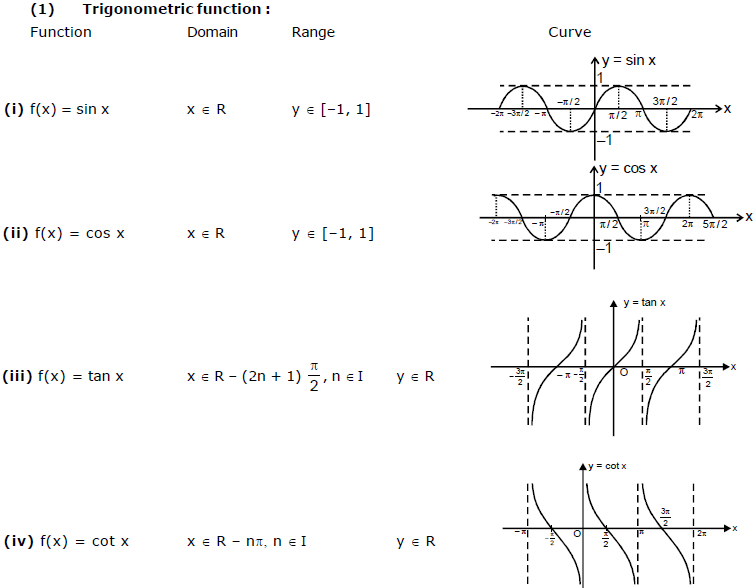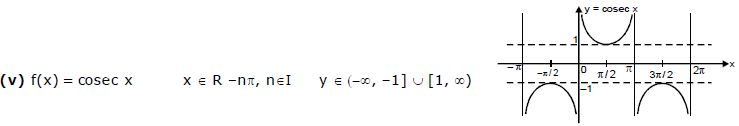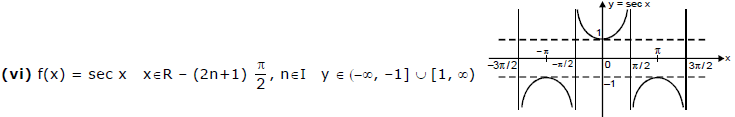(2) Polynomial Function :

f(x) = a0xn + a1xn - 1 + a2 xn-2 +.......+ an

where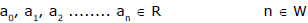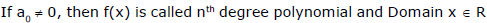(3) Algebraic Function : A function is called an algebraic function. If it can be constructed using algebraic operations such as additions, subtractions, multiplication, division taking roots etc.

All polynomial functions are algebraic but converse is not true.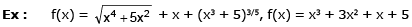Remark : Function which are not algebraic are called as TRANSCENDENTAL FUNCTION.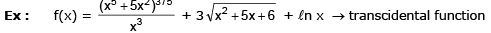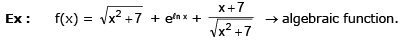(4) Rational Function : It is a function of form f(x) = g(x)/h(x )where g(x) & h(x) are poly. function and h(x) ≠ 0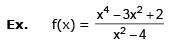Offer running on EduRev: Apply code STAYHOME200 to get INR 200 off on our premium plan EduRev Infinity!

## Mathematics (Maths) Class 11

158 videos|186 docs|161 tests

,

,

,

,

,

,

,

,

,

,

,

,

,

,

,

,

,

,

,

,

,

;